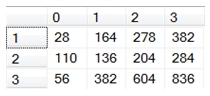# SQL Server matrix muliplication

MATMULT

Updated: 31 January 2012

Use the scalar function MATMULT to calculate the product of two matrixes or to multiply a matrix by a constant.
When multiplying two matrices the result is a matrix where the number of rows is equal to the number of rows in the first matrix and the number of columns is equal to the number of columns in the second matrix.
MATMULT expects a string representation of the matrices, with columns separated by commas and rows separated by semi-colons.
Syntax
SELECT [wctMath].[wct].[MATMULT] (
<@Matrix1, nvarchar(max),>
,<@Matrix2, nvarchar(max),>)
Arguments
@Matrix1
a string representation of a matrix.
@Matrix2
a string representation of a matrix.
Return Types
[nvarchar](max)
Remarks
·         When multiplying two matrices, the number of columns in @Matrix1 must be equal to the number of rows in @Matrix2 or an error will be returned.
·         The string representations of @Matrix1 and @Matrix2 must only contain numbers, commas to separate the columns, and semi-colons to separate the rows.
·         Consecutive commas will generate an error.
·         Consecutive semi-colons will generate an error.
·         Non-numeric data between commas will generate an error
·         Non-number data between semi-colons will generate an error
·         To convert non-normalized data to a string format, use the Matrix2String or the Matrix2String_q function.
·         To convert normalized data to a string format, us the NMatrix2String or the NMatrix2String_q function.
·         To convert the string result to a table, us the table-valued function MATRIX.
·         To perform matrix multiplication directly on normalized data, use the table-valued functions MMULTN or MMULTN_q
·         To perform matrix multiplication directly on non-normalized data, us the table-valued functions MMULT or MMULT_q.
Examples
Let’s assume that we had the following matrices, A & B, and we wanted to multiply A * B:
A = [5,6,7,8;9,10,-11,12;16,15,14,13]
B = [1,8,9,13;2,7,10,14;-3,6,11,15;4,5,12,16]
We could enter the following SQL to perform the multiplication.
DECLARE @A as varchar(max)
DECLARE @B as varchar(max)

SET @A = '5,6,7,8;9,10,-11,12;16,15,14,13'
SET @B = '1,8,9,13;2,7,10,14;-3,6,11,15;4,5,12,16'

SELECT wct.MATMULT(@A,@B) as MATMULT
This produces the following result.
MATMULT
---------------------------------------------
28,164,278,382;110,136,204,284;56,382,604,836
The matrices do not have to assigned to variables before being passed into the MATMULT function; the strings can be passed in directly.
SELECT wct.MATMULT('5,6,7,8;9,10,-11,12;16,15,14,13','1,8,9,13;2,7,10,14;-3,6,11,15;4,5,12,16') as MATMULT
This produces the following result.
MATMULT
---------------------------------------------
28,164,278,382;110,136,204,284;56,382,604,836
In this example, the matrix values are stored on a table in the database and are converted to a string value using the MATRIX2STRING function.
/* Put A into a table */
SELECT *
INTO #A
FROM (
SELECT 5,6,7,8 UNION ALL
SELECT 9,10,-11,12 UNION ALL
SELECT 16,15,14,13
) A(xo,x1,x2,x3)
/* Put B into a table */
SELECT *
INTO #B
FROM (
SELECT 1,8,9,13 UNION ALL
SELECT 2,7,10,14 UNION ALL
SELECT -3,6,11,15 UNION ALL
SELECT 4,5,12,16
) B(x0,x1,x2,x3)
/* Do the matrix multiplication */
SELECT wct.MATMULT(wct.MATRIX2STRING('#A','*','',NULL), wct.MATRIX2STRING('#B','*','',NULL)) as MATMULT
This produces the following result.
MATMULT
---------------------------------------------
28,164,278,382;110,136,204,284;56,382,604,836
If we wanted to return the matrix product as a normalized table, we can use the table-valued function MATRIX to do that.
SELECT *
FROM wct.MATRIX((SELECT wct.MATMULT('5,6,7,8;9,10,-11,12;16,15,14,13','1,8,9,13;2,7,10,14;-3,6,11,15;4,5,12,16')))
This produces the following result.
RowNum      ColNum              ItemValue
----------- ----------- ----------------------
0           0                     28
0           1                    164
0           2                    278
0           3                    382
1           0                    110
1           1                    136
1           2                    204
1           3                    284
2           0                     56
2           1                    382
2           2                    604
2           3                    836

If we wanted to return the results in matrix form, we can use the PIVOT function, though this requires knowing the number of columns returned by the function (which is the number of columns in the second array).

SELECT ,,,
FROM (
SELECT *
FROM wct.MATRIX((SELECT wct.MATMULT('5,6,7,8;9,10,-11,12;16,15,14,13','1,8,9,13;2,7,10,14;-3,6,11,15;4,5,12,16')))
) M PIVOT (
MAX(ItemValue)
FOR colnum IN (,,,)
) AS pvt
ORDER BY rownum
This produces the following result.In this example we will multiply the matrix by 5.
DECLARE @A as varchar(max)

SET @A = '5,6,7,8;9,10,-11,12;16,15,14,13'

SELECT wct.MATMULT(@A,'5') as MATMULT
This produces the following result.
MATMULT
------------------------------------
25,30,35,40;45,50,-55,60;80,75,70,65

### SupportCopyright 2008-2021 Westclintech LLC         Privacy Policy        Terms of Service